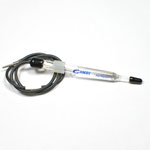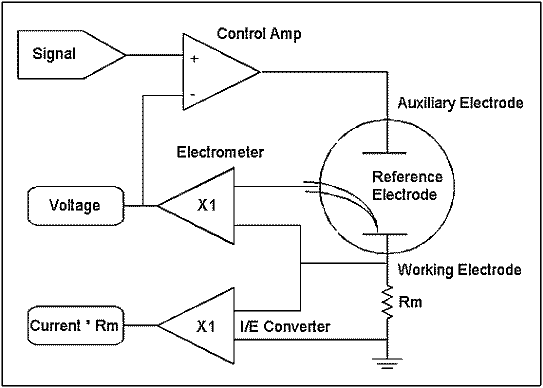Potentiostat Fundamentals

Introduction

A potentiostat is an electronic instrument that controls the voltage difference between a Working Electrode and a Reference Electrode. Both electrodes are contained in an electrochemical cell. The potentiostat implements this control by injecting current into the cell through an Auxiliary, or Counter, electrode.

In almost all applications, the potentiostat measures the current flow between the Working and Counter electrodes. The controlled variable in a potentiostat is the cell potential and the measured variable is the cell current.

Prerequisites

This Application Note may be difficult to follow unless you have some familiarity with electrical terms such as voltage, current, resistance, frequency, and capacitance. If your knowledge in this area is lacking, we suggest review of a basic electronics or physics book.

Electrodes

A potentiostat requires an electrochemical cell with three electrodes.

Working Electrode

The Working Electrode is the electrode where the potential is controlled and where the current is measured. For many physical electrochemistry experiments, the Working Electrode is an “inert” material such as gold, platinum, or glassy carbon. In these cases, the Working Electrode serves as a surface on which the electrochemical reaction takes place.

In corrosion testing, the Working Electrode is a sample of the corroding metal. Generally, the Working Electrode is not the actual metal structure being studied. Instead, a small sample is used to represent the structure. This is analogous to testing using weight-loss coupons. The Working Electrode can be bare metal or coated.

For batteries, the potentiostat is connected directly to the anode or cathode of the battery.

Reference ElectrodeSilver/silver chloride (Ag/AgCl) reference electrode

The Reference Electrode is used to measure the Working Electrode potential. A Reference Electrode should have a constant electrochemical potential as long as no current flows through it.

The most common lab Reference Electrodes are the saturated calomel electrode (SCE) and the silver/silver chloride (Ag/AgCl) electrodes (Figure 2). In field probes, a pseudo-reference (a piece of the Working Electrode material) is often used

Counter (Auxiliary) Electrode

The Counter, or Auxiliary, Electrode is a conductor that completes the cell circuit.

The Counter Electrode in lab cells is generally an inert conductor like platinum or graphite. In field probes, it is generally another piece of the Working Electrode material. The current that flows into the solution via the Working Electrode leaves the solution via the Counter Electrode.

The electrodes are immersed in an electrolyte (an electrically conductive solution). The collection of the electrodes, the solution, and the container holding the solution are referred to as an electrochemical cell.

Simplified Schematic

A simplified schematic of a Gamry Instruments’ potentiostat is sketched in Figure 3.Simple schematic of a potentiostat.

The electronic circuit consists of four blocks discussed in turn. If you are not familiar with electronics, don’t despair; you can still learn from the information that follows.

In this schematic, ×1 on an amplifier indicates that the amplifier is a unity-gain differential amplifier. The output voltage of this circuit is the difference between its two inputs.

The blocks labeled Voltage and Current × Rm are the voltage and current signals that are sent to the system A/D converters for digitization.

The Electrometer

The electrometer circuit measures the voltage difference between the reference and working electrodes. Its output has two major functions: it is the feedback signal in the potentiostat circuit, and it is the signal that is measured whenever the cell voltage is needed.

An ideal electrometer has zero input current and an infinite input impedance. Current flow through the reference electrode can change its potential. In practice, all modern electrometers have input currents close enough to zero that this effect can usually be ignored.

Two important electrometer characteristics are its bandwidth and its input capacitance. The electrometer bandwidth characterizes the AC frequencies the electrometer can measure when it is driven from a low-impedance source. The electrometer bandwidth must be higher than the bandwidth of the other electronic components in the potentiostat.

The electrometer input capacitance and the reference electrode resistance form an RC-filter. If this filter’s time constant is too large, it can limit the effective bandwidth of the electrometer and cause system instabilities. Smaller input capacitance translates into more stable operation and greater tolerance for high impedance reference electrodes.

The I/E Converter

The current-to-voltage (I/E) converter in the simplified schematic measures the cell current. It forces the cell current to flow through a current-measurement resistor, Rm. The voltage drop across Rm is a measure of the cell current.

Cell current in some experiments don’t change much. In other experiments, such as a corrosion experiment, the current can often vary by as much as seven orders of magnitude. You cannot measure current over such a wide range using a single resistor. A number of different Rm resistors can be automatically switched into the I/E circuit. This allows measurement of widely varying currents, with each current measured using an appropriate resistor. An “I/E autoranging” algorithm is often used to select the appropriate resistor values.

The I/E converter’s bandwidth depends strongly on its sensitivity. Measurement of small currents requires large Rm values. Stray (unwanted) capacitance in the I/E converter forms an RC-filter with Rm, limiting the I/E bandwidth. No potentiostat can accurately measure 10 nA at 100 kHz because the bandwidth on this current range is too low to measure a frequency of 100 kHz. This effect becomes very, very important in EIS measurements!

The Control Amplifier

The control amplifier is a servo amplifier. It compares the measured cell voltage with the desired voltage and drives current into the cell to force the voltages to be the same.

Note that the measured voltage is input into the negative input of the control amplifier. A positive perturbation in the measured voltage creates a negative control amplifier output. This negative output counteracts the initial perturbation. This control scheme is known as negative feedback.

Under normal conditions, the cell voltage is controlled to be identical to the signal source voltage.

The control amplifier has a limited output capability. In the case of the Gamry Instruments’ Reference 3000, the control amplifier cannot output more than 32 V or more than 3 A.

The Signal

The signal circuit is a computer-controlled voltage source. It is generally the output of a Digital-to-Analog (D/A) converter that converts computer-generated numbers into voltages.

Proper choice of number sequences allows the computer to generate constant voltages, voltage ramps, and even sine waves at the signal circuit’s output.

When a D/A converter is used to generate a waveform such as a sine wave or a ramp, the waveform is a digital approximation of the equivalent analog waveform, with small voltage steps. The size of these steps is controlled by the resolution of the D/A converter and the rate it at which it is being updated with new numbers.

Galvanostats and ZRAs

You can use a potentiostat as a galvanostat or as a ZRA (Zero-resistance Ammeter). The potentiostat in simplified schematic becomes a galvanostat when the feedback is switched from the cell voltage signal to the cell current signal. The instrument then controls the cell current rather than the cell voltage. The electrometer output can still be used to measure the cell voltage.

A ZRA allows you to force a potential difference of zero volts between two electrodes. The cell current flowing between the electrodes can be measured. A ZRA is often used to measure galvanic corrosion phenomena and electrochemical noise.